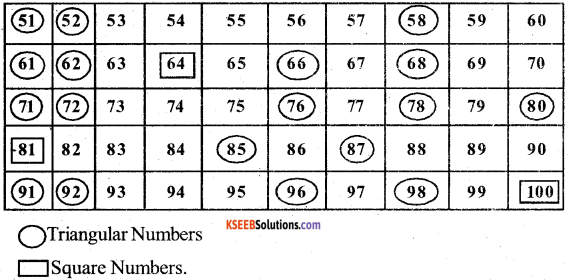# KSEEB Solutions for Class 5 Maths Chapter 10 Patterns

Students can Download Maths Chapter 10 Patterns Questions and Answers, Summary, Notes Pdf, KSEEB Solutions for Class 5 Maths helps you to revise the complete Karnataka State Board Syllabus and score more marks in your examinations.

## Karnataka State Syllabus Class 5 Maths Chapter 10 Patterns

### KSEEB Class 5 Maths Patterns Ex 10.1

I. Solve the following problems:

1. Write the numbers from 1 to 50.

Color the square numbers in red and triangular numbers in blue.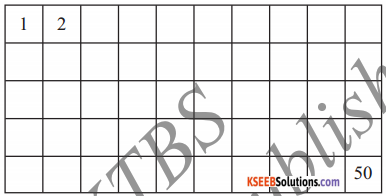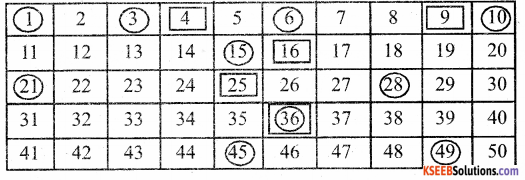Question a.
List the square numbers.
1, 4, 9, 16, 25, 36, 49

Question b.
List the triangular numbers
1, 3, 6, 10, 15, 21. 28, 36, 45Question c.
How many square numbers are there from 1 to 50?
7 Numbers

Question d.
How many triangular numbers are there from 1 to 50?
9 Numbers2. Observe e the given pattern of numbers and fill in the square provided.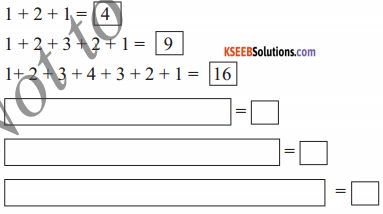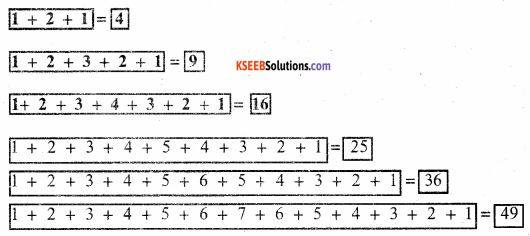3. Express the following square numbers as the sum of consecutive odd numbers. 16, 36, 81, 100, 121, 144, 169, 225
Example : 9 = 1 + 3 + 5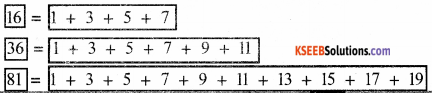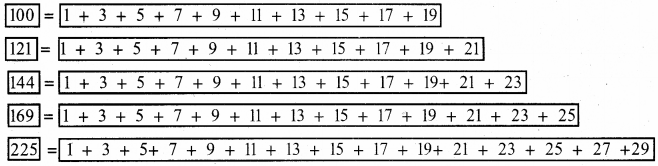4. Fill in the missing odd number and write the square number in the circle.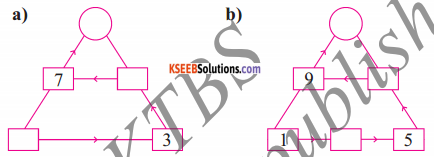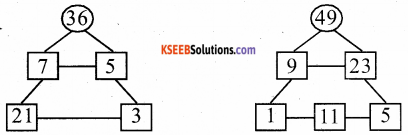5. List the first four square numbers and triangular numbers. Represent them through dot diagrams.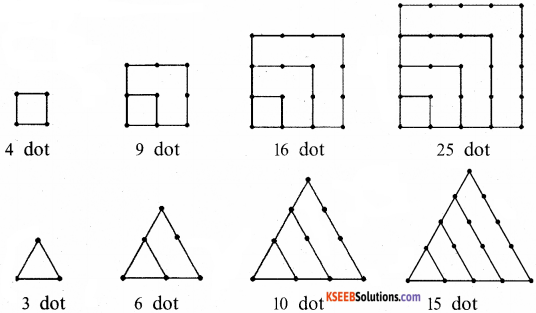6. Here is fun with triangular numbers. First complete the list and then write then write them in the table as shown.
1, 3, 6, 10, → → → 36, 45.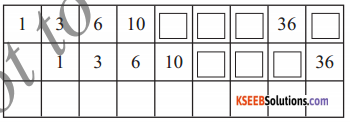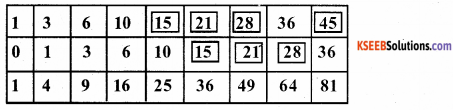Now add them together and write the sum in the bottom row.
What can you say about the numbers formed in this row?
Write your conclusion in the form of a statement.
All numbers are square numbers.7. Observe the given table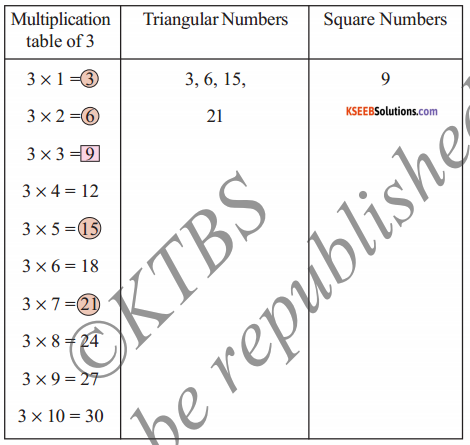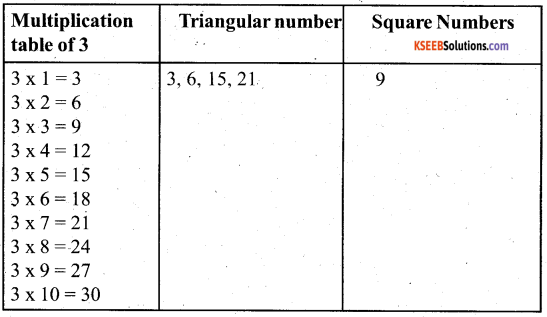Write multiplication tables of 4,5 and 6. In each table, circle the triangular numbers as shown in the example. Draw a box for square numbers. List them in columns as shown.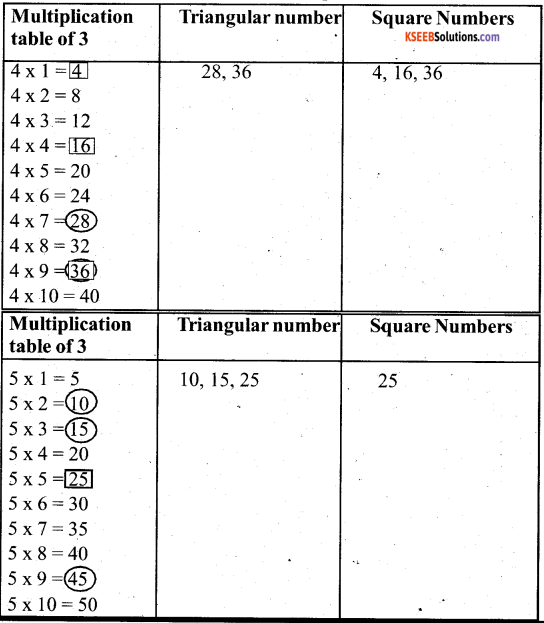Write numbers from 51 to 100. Identify and list triangular numbers and square numbers.